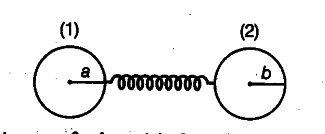# Two charged conducting spheres of radii a and b are connected to each other by a wire

Two charged conducting spheres of radii a and b are connected to each other by a wire. What is the ratio of electric fields at the surfaces of the two spheres? Use the result obtained to explain, why charge density on the sharp and pointed ends of a conductor is higher than on its . flatter portions?

As, the two conducting spheres are connected to each other by a wire, the charge always flows from higher potential to lower potential till both have same potential.As, charge density is inversely proportional to radius. Thus, for flatter portions, tfie radius is more and at pointed ends, radius is less, thus the charge density is more at pointed or sharp ends.